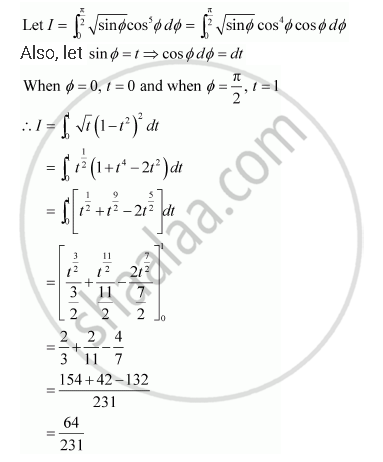Share

# Evaluate the Integrals in Using Substitution Int_0^(Pi/2) Sqrt(Sin Phi) Cos^5 Phidphi - CBSE (Science) Class 12 - Mathematics

ConceptEvaluation of Definite Integrals by Substitution

#### Question

Evaluate the integrals in using substitution

int_0^(pi/2) sqrt(sin phi) cos^5 phidphi

#### SolutionIs there an error in this question or solution?

#### Video TutorialsVIEW ALL 

Solution Evaluate the Integrals in Using Substitution Int_0^(Pi/2) Sqrt(Sin Phi) Cos^5 Phidphi Concept: Evaluation of Definite Integrals by Substitution.
S